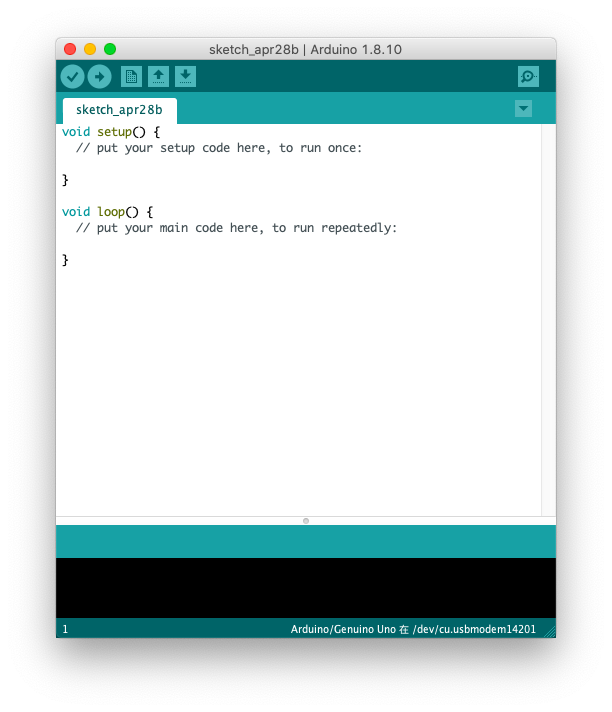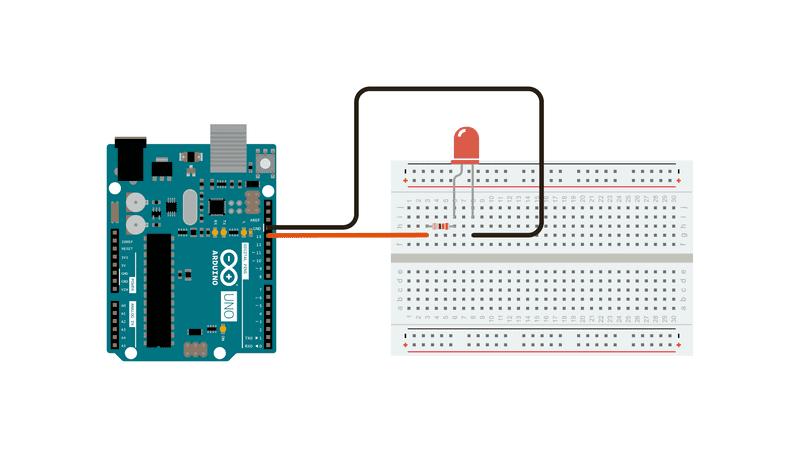# Arduino 程序结构

## 程序框架

Arduino 使用 C/C++ 语言编写程序，但 Arduino 程序的结构与传统 C/C++ 的程序结构又有所不同 —— Arduino 程序中没有 main() 函数。Arduino 程序的基本结构由 setup() 和 loop() 两个函数组成。

• setup()

Arduino 控制器通电或复位后，即会开始执行 setup() 函数中的程序，该程序只会执行一次。通常在这里完成 Arduino 的初始化设置，如初始化变量、初始化串口、配置 I/O 状态、启用软件库等操作。

• loop()

setup() 函数中的程序执行完毕后，Arduino 会接着执行 loop() 函数中的程序，而 loop() 函数是一个死循环，其中的程序会不断地重复运行，使得 Arduino 可以不断工作。通常在这里完成程序的主要功能，如驱动各种模块和采集数据等。

``````void setup()
{
/* 初始化函数，配置管脚、调用库函数 */
}

void loop()
{
/* 连续执行函数内的语句 */
}
``````

## Hello World

Arduino IDE 内置示例程序，包括基本的、数字的、模拟的、控制的、通信的、传感器的、字符串的、存储卡的、显示的、音频的、网络的示例等等。您可在菜单栏中选择“文件”->“示例”中找到它们。

``````/*
Turns an LED on for one second, then off for one second, repeatedly.
*/

// the setup function runs once when you press reset or power the board
void setup() {
// initialize digital pin LED_BUILTIN as an output.
pinMode(LED_BUILTIN, OUTPUT);
}

// the loop function runs over and over again forever
void loop() {
digitalWrite(LED_BUILTIN, HIGH);   // turn the LED on (HIGH is the voltage level)
delay(1000);                       // wait for a second
digitalWrite(LED_BUILTIN, LOW);    // turn the LED off by making the voltage LOW
delay(1000);                       // wait for a second
}
``````

``````#define LED_BUILTIN 13
``````

``````pinMode(LED_BUILTIN, OUTPUT);
``````

``````digitalWrite(LED_BUILTIN, HIGH);
``````

``````delay(1000);
digitalWrite(LED_BUILTIN, LOW);
``````## main 函数解析

``````#include <Arduino.h>

// Declared weak in Arduino.h to allow user redefinitions.
int atexit(void (* /*func*/ )()) { return 0; }

// Weak empty variant initialization function.
// May be redefined by variant files.
void initVariant() __attribute__((weak));
void initVariant() { }

void setupUSB() __attribute__((weak));
void setupUSB() { }

int main(void)
{
init();

initVariant();

#if defined(USBCON)
USBDevice.attach();
#endif

setup();

for (;;) {
loop();
if (serialEventRun) serialEventRun();
}

return 0;
}
``````

loop 函数的循环执行，是通过 for 循环实现的，且每次 loop 结束后，都会进行串口事件判断，也正是因为这种设计，串口事件不能实时响应。